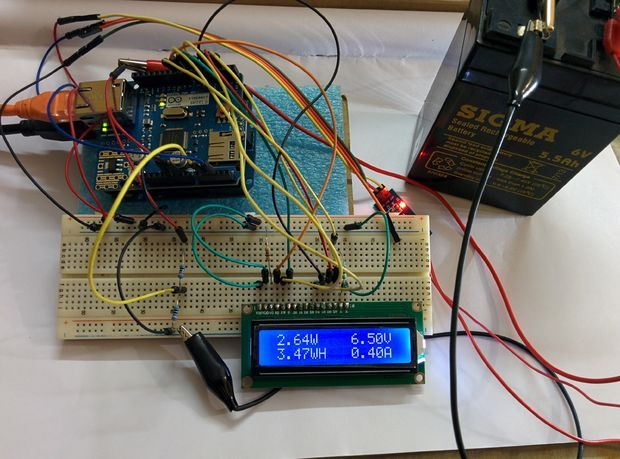# Arduino Energy meter

I belong to a village of Odisha, India where frequent power cut is very common. It hampers the life of every one. During my childhood days continuing studies after dusk was a real challenge. Due to this problem I designed a solar system for my home on a experimental basis. I used a solar panel of 10 Watt ,6V for lighting few bright LEDs. After facing lot of hardships the project was successful. Then I decided to monitor the voltage, current, power & energy involved in the system. This brought the idea of designing an ENERGY METER.I used ARDUINO as the heart of this project because it is very easy to write code in its IDE and there are huge numbers of open source library available in the internet which can be used according to the requirement.I have experimented the project for very small rated (10Watt) solar system but this can be easily modified to use for higher rating system.Feature:
Energy monitoring by
1.LCD display
3. Data logging in a SD card
You can also see my other instructables on

ARDUINO SOLAR CHARGE CONTROLLER (PWM)

## Step 1: Parts Required :

1. ARDUINO UNO (Rev-3)
2. ARDUINO ETHERNET SHIELD

3. 16×2 CHARACTER LCD
4. ACS 712 CURRENT SENSOR
4. RESISTORS (10k ,330ohm)
5. POTENTIOMETER (10K)
6. JUMPER WIRES
7. ETHERNET CABLE (CAT-5E)

## Step 2: Power and Energy

Power :
Power is product of voltage (volt) and current (Amp)
P=VxI
Unit of power is Watt or KW
Energy:
Energy is product of power (watt) and time (Hour)
E= Pxt
Unit of Energy is Watt Hour or Kilowatt Hour (kWh)
From the above formula it is clear that to measure Energy we need three parameters
1. Voltage
2. Current
3. Time

READ  Large 7-Segment Clock, Part I

## Step 3: Voltage Measurement

Voltage is measured by the help of a voltage divider circuit.As the ARDUINO analog pin input voltage is
restricted to 5V I designed the voltage divider in such a way that the output voltage from it should be less than 5V.My battery used for storing the power from the solar panel is rated 6v, 5.5Ah.So I have to step down this 6.5v to a voltage lower than 5V.
I used R1=10k and R2 =10K. The value of R1 and R2 can be lower one but the problem is that when resistance is low higher current flow through it as a result large amount of power (P = I^2R) dissipated in the form of heat. So different resistance value can be chosen but care should be taken to minimize the power loss across the resistance.

Vout=R2/(R1+R2)*Vbat
Vbat=6.5 when fully charged
R1=10k and R2=10k
Vout=10/(10+10)*6.5=3.25v which is lower than 5v and suitable for ARDUINO analog pin
NOTE
I have shown 9 Volt battery in bared board circuit is just for example to connect the wires.But the actual battery I used is a 6 Volt, 5.5Ah lead acid battery.
Voltage Calibration:
When battery is fully charged (6.5v) we will get a Vout=3.25v and lower value for other lower battery voltage.
AEDUINO ADC convert Analog signal to corresponding digital approximation .
When the battery voltage is 6.5v I got 3.25v from the voltage divider and sample1 = 696 in serial monitor ,where sample1 is ADC value corresponds to 3.25v

For better understanding I have attached the real time simulation by 123D.circuit for voltage measurement
Calibration:
3.25v equivalent to 696
1 is equivalent to 3.25/696=4.669mv
Vout = (4.669*sample1)/1000 volt
Actual battery voltage = (2*Vout) volt
ARDUINO CODE:
// taking 150 samples from voltage divider with a interval of 2sec and then average the samples data collected for(int i=0;i<150;i++)
delay (2);
sample1=sample1/150;
voltage=4.669*2*sample1/1000;

READ  Flexible and transparent OLEDs at LG

## Step 4: Current Measurement

For current measurement I used a Hall Effect current sensor ACS 712 (20 A).There are different current range ACS712 sensor available in the market, so choose according to your requirement. In bread board diagram I have shown LED as a load but the actual load is different.
WORKING PRINCIPLE :
The Hall Effect is the production of a voltage difference (the Hall voltage) across an electrical conductor, transverse to an electric current in the conductor and a magnetic field perpendicular to the current.
The data sheet of ACS 712 sensor is found here
From Data Sheet
1. ACS 712 measure positive and negative 20Amps, corresponding to the analog output 100mV/A
2. No test current through the output voltage is VCC / 2 =5v/2=2.5V
Calibration:
Analog read produces a value of 0-1023, equating to 0v to 5v
So Analog read 1 = (5/1024) V =4.89mv
Value = (4.89*Analog Read value)/1000 V
But as per data sheets offset is 2.5V (When current zero you will get 2.5V from the sensor’s output)
Actual value = (value-2.5) V
Current in amp =actual value*10

ARDUINO CODE:
// taking 150 samples from sensors with a interval of 2sec and then average the samples data collected
for(int i=0;i<150;i++)
delay(2);
sample2=sample2/150;
val =(5.0*sample2)/1024.0;
actualval =val-2.5; // offset voltage is 2.5v
amps =actualval*10;

For more detail: Arduino Energy meter

#### This Post / Project can also be found using search terms:

• arduino energy meter code
• energy meter using arduino
• smart energy meter using arduino
• energy meter code
READ  Arduino IDE released for ESP8266